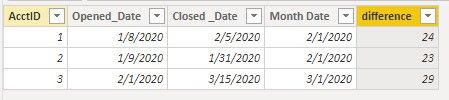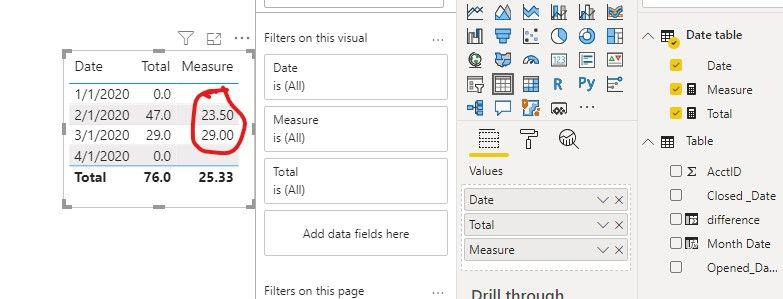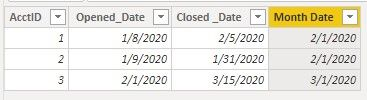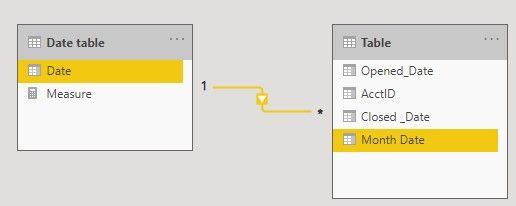cancel
Showing results for
Did you mean:Helper I

## Average Age of Accounts by Month

Hello,  For simplicity, I have a table

 AcctID Opened_Date Closed _Date 1 1/8/2020 2/5/2020 2 1/9/2020 1/31/2020 3 2/1/2020 3/15/2020

I need to caluclate the age of the account at the beginning of every month of 2020

Result I need for my 100,000s records

 Date Avg Age at Date (Logic for bi community help) 1/1/2020 0 (None are Open then) 2/1/2020 23.5 (Avg of 1 and 2 because both are open at 2/1) 3/1/2020 29 (BC 1 and 2 are closed and Account 3 is open and 29 days old) 4/1/2020 0 (bc account 3 has closed by 4/1)

1 ACCEPTED SOLUTIONCommunity Support

Hi @billuran ,

We have a little confused about your issue, what the total avg age?

Is it the Total day of the current month divided by the ID of the current month?

For example, the 2020/2/1 has two ID, the total day is 23 + 24 = 47. So the result is 47 / 2 = 23.5

If yes, you can refer the following steps.

1. Create a calculate column named Month date.

``Month Date = CALCULATE(MIN('Date table'[Date]),FILTER('Date table','Date table'[Date]>'Table'[Opened_Date]))``2. Then create the difference days column.

``difference = DATEDIFF('Table'[Opened_Date],'Table'[Month Date],DAY)``3. Then we can create the Total measure and result measure.

``Total = SUM('Table'[difference])+0``

``````Measure =
var _amount = DISTINCTCOUNT('Table'[AcctID])
return
DIVIDE([Total],_amount)``````If it doesn’t meet your requirement, could you please show the exact expected result based on the table that you have shared?

Best regards,

Community Support Team _ zhenbw

If this post helps, then please consider Accept it as the solution to help the other members find it more quickly.

BTW, pbix as attached.

6 REPLIES 6Community Support

Hi @billuran ,

We can create a calculate column and a relationship, then create a measure to meet your requirement.

1. Create a calculate column that gets the min month date.

``Month Date = CALCULATE(MIN('Date table'[Date]),FILTER('Date table','Date table'[Date]>'Table'[ Opened_Date]))``2. We can create a one-to-many relationship between table and Date table.3. Then we can create a measure and the result like this,

``Measure = AVERAGEX('Table',DATEDIFF('Table'[ Opened_Date],'Table'[Month Date],DAY))+0``If it doesn’t meet your requirement, could you please show the exact expected result based on the table that you have shared?

Best regards,

Community Support Team _ zhenbw

If this post helps, then please consider Accept it as the solution to help the other members find it more quickly.

BTW, pbix as attached.Helper I

The formula seems to only show the avg age between Month Date, I woudl like the total avg age from Opened to the Month DateCommunity Support

Hi @billuran ,

We have a little confused about your issue, what the total avg age?

Is it the Total day of the current month divided by the ID of the current month?

For example, the 2020/2/1 has two ID, the total day is 23 + 24 = 47. So the result is 47 / 2 = 23.5

If yes, you can refer the following steps.

1. Create a calculate column named Month date.

``Month Date = CALCULATE(MIN('Date table'[Date]),FILTER('Date table','Date table'[Date]>'Table'[Opened_Date]))``2. Then create the difference days column.

``difference = DATEDIFF('Table'[Opened_Date],'Table'[Month Date],DAY)``3. Then we can create the Total measure and result measure.

``Total = SUM('Table'[difference])+0``

``````Measure =
var _amount = DISTINCTCOUNT('Table'[AcctID])
return
DIVIDE([Total],_amount)``````If it doesn’t meet your requirement, could you please show the exact expected result based on the table that you have shared?

Best regards,

Community Support Team _ zhenbw

If this post helps, then please consider Accept it as the solution to help the other members find it more quickly.

BTW, pbix as attached.Community Support

Hi @billuran ,

How about the result after you follow the suggestions mentioned in my original post?

Could you please provide more details or expected result about it If it doesn't meet your requirement?

If you've fixed the issue on your own please kindly share your solution. If the above posts help, please kindly mark it as a solution to help others find it more quickly.

Best regards,

Community Support Team _ zhenbw

If this post helps, then please consider Accept it as the solution to help the other members find it more quickly.Super User

@billuran - Perhaps:

``````Measure =
AVERAGEX(FILTER('Table',[Opened_Date]>MAX([Date])),([Closed Date] - [Opened Date])*1.)``````

Become an expert!: Enterprise DNA
External Tools: MSHGQM
Latest book!:
Mastering Power BI 2nd EditionDAX is easy, CALCULATE makes DAX hard...Super User